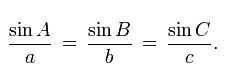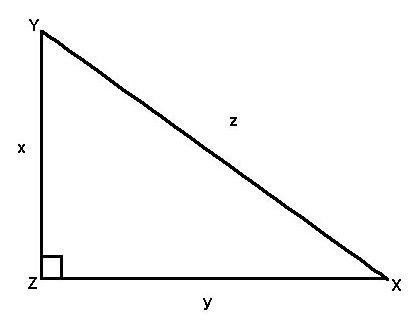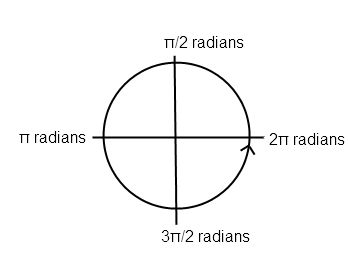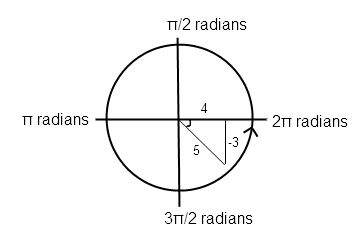# Conquering ACT Trigonometry

Trigonometry is some of hardest math on the ACT (it isn’t tested at all on the SAT) but luckily the ACT trigonometry requires the use of only a few very special formulas! Some of these you may have seen before while some may be entirely new, but you’ll need to memorize all of them to adequately prepare for the test.
Sine = Opposite / Hypotenuse
These are the three most basic trig identities. In plain English, they mean that if you are looking for the “sine” of a certain angle for example, you would divide the length of side opposite that angle by the length of the hypotenuse of the triangle. It’s important to remember that the “opposite” and “adjacent” sides change depending on which angle you are using, so always think of it from the point of view of the angle. The easiest way to remember these basic identities is the acronym SOHCAHTOA.
You will definitely encounter questions that require you to use SOHCAHTOA, and you may encounter questions that ask about reciprocal trig identities. Each of the three basic trig identities has a corresponding reciprocal trig identity:
Cosecant = Hypotenuse / Opposite
Notice how Sine and Cosecant are the same except the numerator and denominator is flipped. That’s what we mean by reciprocal. It’s easy to remember that “tangent” and “cotangent” are reciprocals since they sound so much alike, but how what about the other two? I once had a math teacher who used, “Co-co no go” as a mnemonic device to help my high school class remember. What he meant was that your brain may think that “cosine” and “cosecant” are reciprocals since they both begin with the prefix “co-“ but that isn’t true. “Sine” goes with “cosecant” and “cosine” goes with “secant.”
Finally, there are two more trig equations that appear from time to time in ACT questions:
Sinθ + Cosθ = 1The second equation is referred to as the law of sines (where a, b, and c are the sides of the triangle and A, B and C are the opposite angles).
Let’s try a few practice problems!
For right triangle XYZ, what is  cos X?A.  x/y
B. z/y
C. x/z
D. y/x
E. y/z
We remember from SOHCAHTOA that cosine = adjacent / hypotenuse. From the point of view of angle X, y is the adjacent side and z is the hypotenuse. Therefore the answer must be E.
If cos theta = 4/5 and 3π/2 < theta < 2π, then sin theta =?
A  –3/4
B –3/5
C  3/5
D  4/5
E  5/4

For this question we have two ways to solve: draw and label the triangle, or use the formula Sinθ + Cosθ = 1. For both, we first need to review our understanding of radians.A radian is simply another way of measuring an angle. We are used to measuring and expressing angles in degrees. Some harder problems on the ACT will use radians instead of degrees.
There are 2π radians in one circle. Each point on a circle corresponds to a certain number of radians.
By telling us that angle theta is between 3π/2 and 2π, we know that the angle must be in the 4th quadrant of the circle.
We know cosine = adjacent / hypotenuse, so we can label those two sides 4 and 5. Since we’ve been studying our Pythagorean triplets we know that the third side must be 3! But because it’s in the 4th quadrant, we can see that it will be a -3.
Sin theta = opposite / hypotenuse = -3/5. The correct answer is B.If we had used the equation Sinθ + Cosθ = 1, we would have squared the cosine 4/5 to become cosine 16/25 and then solved for sine. It would still have required us to know, however, that the 3 is negative since it’s in the 4th quadrant.

Tags: